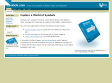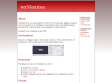Public marks with tag symbolic http://blogmarks.net/marks/tag/symbolic Symbols.com - Home http://symbols.com/ 2006-04-26T08:14:52Z terrybain book, symbol, symbols, directory, symbolic]]> Axiom Computer Algebra System - Summary [Savannah] http://savannah.nongnu.org/projects/axiom/ Axiom is a general purpose Computer Algebra system. It is useful for doing mathematics by computer and for research and development of mathematical algorithms. It defines a strongly typed, mathematically correct type hierarchy. It has a programming langua 2006-01-06T07:32:26Z YukuanMark freeware, scientific, cas, algebra, symbolic#### Axiom Computer Algebra System - Summary [Savannah]by YukuanMark
Axiom is a general purpose Computer Algebra system. It is useful for doing mathematics by computer and for research and development of mathematical algorithms. It defines a strongly typed, mathematically correct type hierarchy. It has a programming langua
]]>
wxMaxima http://wxmaxima.sourceforge.net/ wxMaxima is a cross platform GUI for the computer algebra system maxima based on wxWidgets. It provides menu and dialog based interface for maxima and a nice display of math output. 2006-01-05T20:28:14Z YukuanMark freeware, scientific, maxima, cas, algebra, symbolic#### wxMaximaby YukuanMark
wxMaxima is a cross platform GUI for the computer algebra system maxima based on wxWidgets. It provides menu and dialog based interface for maxima and a nice display of math output.
]]>
Maxima - A GPL CAS based on DOE-MACSYMA http://maxima.sourceforge.net/index.shtml Maxima is a system for the manipulation of symbolic and numerical expressions, including differentiation, integration, Taylor series, Laplace transforms, ordinary differential equations, systems of linear equations, and vectors, matrices, and tensors. Max 2006-01-05T19:07:08Z YukuanMark freeware, scientific, cas, algebra, mathmatica, symbolic#### Maxima - A GPL CAS based on DOE-MACSYMAby YukuanMark & 2 others
Maxima is a system for the manipulation of symbolic and numerical expressions, including differentiation, integration, Taylor series, Laplace transforms, ordinary differential equations, systems of linear equations, and vectors, matrices, and tensors. Max
]]>